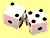## Generating Uniform Distribution using Pseudo Random

Primitive of random number for Monte Carlo Simulation is distributed according to Uniform distribution with PDF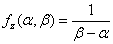,

where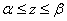is the boundary of the distribution. The inverse distribution function is given by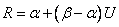The figure below show the visual distribution of MS Excel Uniform Random number. Its clearly distributed according to standard uniform distribution.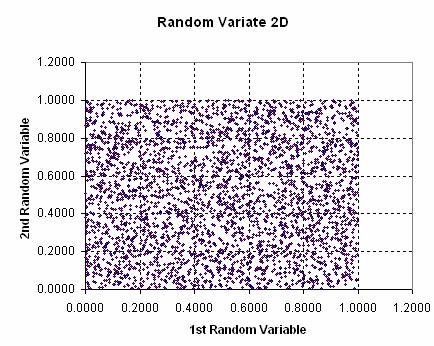The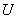must be distributed as standard uniform distribution with range zero to one.

Just in case you need to generate it by yourselves, consider the following explanation. The most widely used of pseudo random generator is to produce standard uniform distributionusing Linear Congruential Generator of Lehmer introduce in 1951: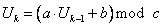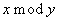returns the remainder of division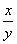. The formula gives a sequence of integers, all different numbers, up to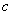elements. To make longest sequence, we make this value as large as possible. We can set this value as the number of storage for integer variables. Suppose we use 32 bits, then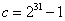. Most commonly use constants is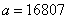and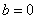.

The seed value of pseudorandom represents the start of sequence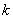. To make it random usually we need to randomize the initial seed.

Most of programming languages have random number function to generate uniform distribution. Below are a few lists of programming language or software and its pseudorandom number. Most of random number generated are in the interval of 0 and 1 (except C/C++ which generate integer random number)

 Programming Language/Software PseudoRandom number function Note Visual Basic or Visual Basic for Application (VBA) Rnd( seed) Use Randomize to initialize seed Matlab rand( matrix_size ) Generate a matrix of random numbers MathCad rnd(x) Random number between 0 and x Visual C/C++ rand() It generates integer 0 to RAND_MAX rather than number between 0 and 1; Include , use srand( seed ) to initialize Java java.lang.Math Visual FoxPro Rand ( seed ) Use seed value first time only Mathematica Random[ type , range ] Use SeedRandom[ seed ] to reset the seed MS Excel worksheet = RAND ()

Preferable reference for this tutorial is

Teknomo, Kardi. Monte Carlo Simulation Tutorial. http://people.revoledu.com/kardi/ tutorial/Simulation